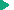GUI for .NET Programming
Using .NET data types in ABL : Accessing and using .NET arrays

# Accessing and using .NET arrays

All .NET arrays are reference type objects that inherit from the .NET abstract class, System.Array, as shown in the following ABL class hierarchy:
 Progess.Lang.Object   <----------------------------- ABL root class   System.Object       <----------------------------- .NET root class     System.Array     <----------------------------- .NET array base class       "ElementType[]" <----------------------------- .NET array class
A .NET array object can represent an array of any .NET value or reference type, including another .NET array type, and with any number of dimensions (starting at one, of course).
The type name of any .NET array type, regardless of extent, is the type name of its elements (ElementType) appended with a pair of square brackets ([]). If the brackets are empty, the type name specifies a one-dimensional array. If the brackets contain commas (for example, [,,]), the type name specifies an additional dimension for each comma. In ABL, you must also surround this type name within quotes to allow the special characters ([,]) in the name, as in the example class hierarchy ("ElementType[]").
For .NET arrays of a primitive type (for example, C# int), the same array type can be represented using the primitive type in a given .NET language (for example, C# int[]) or the equivalent object type alias (for example, System.Int32[]). (For information on the .NET object type aliases for .NET primitive types, see Table 10.) However, where in C# you can reference the same array type as int[] or as System.Int32[], in ABL you can only reference this array type as "System.Int32[]", using the alias object type name. In fact, where in ABL you must access any .NET scalar primitive type (for example, System.Int32) as its equivalent ABL primitive type (for example, INTEGER), you can only reference a .NET primitive type array as a .NET array object in ABL. For example, you cannot reference a "System.Int32[]" array type in ABL as "INTEGER[]", but only as "System.Int32[]".
You can similarly reference the type of any .NET array object, such as "System.Drawing.Point[]", which is a one-dimensional array of .NET graphical point objects, or "System.Drawing.Point[,,]", which is a three-dimensional array of the same object type. .NET actually supports a variety of different kinds of array object types, including jagged arrays, that you can access in ABL. For more information, see the "Arrays Tutorial" in the C# Programmer's Reference on MSDN.
Note that you do not specify the extent (size) for each dimension of a .NET array object as part of its type name. Instead, these values are set when the array object, itself, is created, whether in the .NET or ABL context.
The following sections provide more information on accessing and using .NET arrays in ABL:Accessing .NET arraysArray mapping: conversion between ABL arrays and .NET arraysArray assignmentExample: Mapping an ABL array to a .NET arrayExample: Accessing a .NET arrayAccessing .NET arraysArray mapping: conversion between ABL arrays and .NET arraysArray assignmentExample: Mapping an ABL array to a .NET arrayExample: Accessing a .NET array